A pseudoenergy wave-activity relation for ageostrophic and non-hydrostatic moist atmosphere*
Institute of Atmospheric Physics, Chinese Academy of Sciences, Beijing 100029, China

Corresponding author. E-mail: rlk@mail.iap.ac.cn

*Project supported by the National Basic Research Program of China (Grant No. 2013CB430105), the Key Program of the Chinese Academy of Sciences (Grant No. KZZD-EW-05), the National Natural Science Foundation of China (Grant No. 41175060), and the Project of CAMS, China (Grant No. 2011LASW-B15).

Abstract

By employing the energy-Casimir method, a three-dimensional virtual pseudoenergy wave-activity relation for a moist atmosphere is derived from a complete system of nonhydrostatic equations in Cartesian coordinates. Since this system of equations includes the effects of water substance, mass forcing, diabatic heating, and dissipations, the derived wave-activity relation generalizes the previous result for a dry atmosphere. The Casimir function used in the derivation is a monotonous function of virtual potential vorticity and virtual potential temperature. A virtual energy equation is employed (in place of the previous zonal momentum equation) in the derivation, and the basic state is stationary but can be three-dimensional or, at least, not necessarily zonally symmetric. The derived wave-activity relation is further used for the diagnosis of the evolution and propagation of meso-scale weather systems leading to heavy rainfall. Our diagnosis of two real cases of heavy precipitation shows that positive anomalies of the virtual pseudoenergy wave-activity density correspond well with the strong precipitation and are capable of indicating the movement of the precipitation region. This is largely due to the cyclonic vorticity perturbation and the vertically increasing virtual potential temperature over the precipitation region.

Keyword: 92.60.N–; 92.60.Nv; 92.60.hk; energy-Casimir method; pseudoenergy; wave-activity relation; wave-activity density
1. Introduction

The wave– flow interaction is an important subject of atmospheric dynamics. The flow is referred to as the basic-state flow and the wave is a deviation from the basic-state flow. Their interaction includes two aspects: the feedback to the basic-state flow from the wave, which is described by the E– P flux theory,  and the forcing to the wave by the basic-state flow, which is presented by the wave-activity relation. Because the basic-state flow and the wave vary with different temporal scales, the two aspects can be investigated individually. The wave-activity density, which is quadratic (or of higher order) in the disturbance fields, can be a conserved quantity at the small-amplitude limit.[11, 12] Generally, the wave-activity density satisfies the following wave-activity relation in flux form: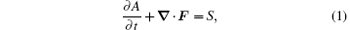where A and F are the wave-activity density and the wave-activity flux, respectively, and S is the source or sink. In the absence of diabatic heating and turbulent dissipation, S tends to be zero, and the above equation becomes conserved. Then, the tendency of the wave-activity density is controlled by the convergence or divergence of the wave-activity flux. Many studies have shown that the wave-activity relation provides a theory that underlies the interaction between the basic state flow and the wave.

Popular methods to construct such a relation include the momentum-Casimir and the energy-Casimir methods, which were used by Arnol’ d and others to prove stability theorems for some conservation flows. McIntyre and Shepherd,  Haynes,  and Scinocca et al. extended these methods to investigate the wave-activity relations for small-amplitude and finite-amplitude disturbances to parallel and nonparallel flows. Ran and Gao employed the momentum-Casimir method to construct the pseudomomentum wave-activity relation for dry air in the nonhydrostatic dynamic framework. The wave– flow interaction was theoretically examined by Ran and Boyd, and its two aspects were linked via the basic-state diabatic heating.

Previous investigations of the wave– flow interaction have mostly been confined to large-scale systems. However, the severe precipitations leading to flood disasters are often associated with the meso-scale systems, and for some time have also attracted much attention. Recently, wave-activity relations were also applied to heavy-rainfall event diagnosis. Ran et al. analyzed the dynamic processes responsible for precipitation during the landfall of typhoon Wipha in terms of the wave-activity density. Since the wave-activity density is capable of describing the typical vertical structure of dynamic and thermodynamic fields, it presents anomalies in the middle and lower troposphere over the strong precipitation area. Chu et al. developed the potential shear wave-activity relation and investigated the meso-scale features in typhoon Morakot’ s (2009) precipitation.

In previous studies of the wave-activity relation, moisture was not explicitly taken into account. The real atmosphere, however, is not truly dry. It contains moisture and is often saturated when heavy rainfall occurs. Thus, it is quite necessary to extend the previous study of Ran and Gao,  and derive a new wave-activity relation involving moisture to diagnose the energetics of meso-scale systems which have interactions with the large-scale basic flow in real moist atmosphere. To realize this, the present paper employs the energy-Casimir method to derive a three-dimensional non-hydrostatic virtual pseudoenergy wave-activity relation in the presence of various hydrometeors and related moist processes. The governing equations are presented in Section 2. The virtual pseudoenergy wave-activity relation for moist atmosphere is derived in Section 3. The results are summarized in Section 4.

2. The virtual pseudoenergy wave-activity relation

Consider a diabatic, viscid, compressible, and moist atmosphere. The governing equations on β -plane in Cartesian coordinates (x, y, z, t) are given by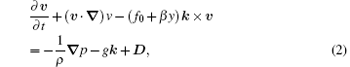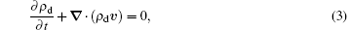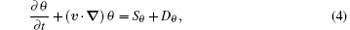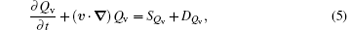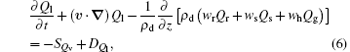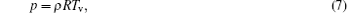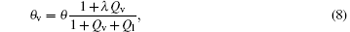where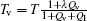is the virtual temperature; θ v is the virtual potential temperature; T is the temperature; λ = 1.608 is a constant; v = (u, v, w) is the three-dimensional velocity; p is the pressure; ρ is the total density of moist atmosphere, which is the sum of dry-air density ρ d, water-vapor density ρ v, cloud-water density ρ c, rain-water density ρ r, ice density ρ i, snow density ρ s, and graupel density ρ g; Qv is the water-vapor mixing ratio; Ql is the mixing ratio sum of cloud water Qc, rain water Qr, ice Qi, snow Qs, and graupel Qg; wr, ws, and wh are the terminal velocities of rain water, snow, and graupel, respectively; θ = T(1000/p)R/cp is the potential temperature; R is the dry-air constant; cp is the specific heat at constant pressure; g is the gravitational acceleration; f0 is the Coriolis parameter; β is the Rossby parameter; and, k is the unit vector in the vertical direction. In addition, Sθ is the diabatic heating; SQv is the source or sink for Qv; and, Du, Dv, Dw, Dθ , DQv, and DQl denote the dissipations of momentum, potential temperature, and water substances, respectively. As an equation system involving water substances, equations  (2)– (8) have been extensively employed in numerical models such as the weather research and forecasting model (WRF),  the advanced region prediction system (ARPS),  and the cloud-resolving model.

By using Eqs.  (3), (5), and (6), one obtains the continuity equation of total mass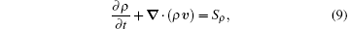where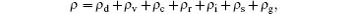and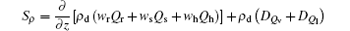is the mass forcing composed by fallouts of Qr, Qi, and Qg at their respective terminal speeds and dissipations of water substances. Qiu et al. examined the significance of mass forcing and found that the mass forcing is important in the numerical simulations of heavy rainfall. The mass forcing resulting from heavy rainfall may cause the anomaly of moist potential vorticity. These studies suggest that the effect of mass forcing on heavy precipitation is not negligible.

The total energy equation is given by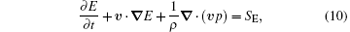where E = (u2 + v2 + w2)/2 + gz + cvTv is the virtual energy density, which is the sum of kinetic energy, potential energy, and virtual internal energy cvTv, and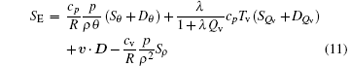is the source or sink for the energy density. The right-hand side terms in Eq.  (11) stand for the contributions of thermal source and dissipation, moisture source and dissipation, momentum dissipations, and mass forcing, respectively. For adiabatic, inviscid, and dry atmosphere, equation (10) is a conservation equation of energy.

By taking moisture into account, the virtual potential vorticity (VPV) is expressed as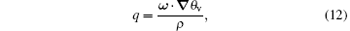where ω = (∂ w/∂ y − ∂ v/∂ z, ∂ u/∂ z − ∂ w/∂ x, ∂ v/∂ x − ∂ u/∂ y + f0 + β y) is the absolute vorticity. The tendency equation of VPV is written as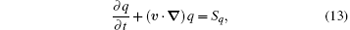where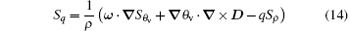is the source or sink of VPV, and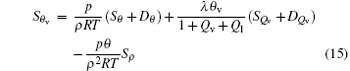is the source or sink of θ v, which contains thermal source and dissipation, moisture source and dissipation, and mass forcing. In the absence of a source or sink for virtual potential temperature, dissipations and mass forcing, VPV satisfies a local conservation equation.

Following Haynes,  we exploit the Casimir function C = C(q, θ v), a single-valued algebraic function of q and θ v. In the conservative case, C is constant following the fluid motion. It satisfies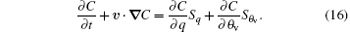After adding the energy equation  (10) to the Casimir equation  (16) and using the total continuity equation  (9), one can obtain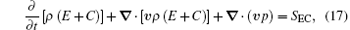where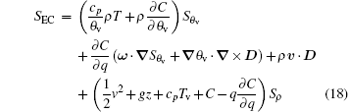is the source or sink.

Based on Eq.  (17), we now use the energy-Casimir method to construct the virtual pseudoenergy wave-activity relation for a moist atmosphere. The Casimir function C is chosen to make the difference between ρ (E + C) and its basic state equal to the divergence of a flux plus a second-order wave quantity and a basic-state quantity. As pointed out by Scinocca and Shepherd,  the energy-Casimir method requires the basic states to be stationary.

Before further derivation, it is necessary to discuss the basic states. Let (u0, v0, p0, T0, ρ 0, θ 0, θ v0, qv0, q0) denote the basic states of (u, v, p, T, ρ , θ , θ v, qv, q), and take the basic-state values of w and ql to be zero. These basic-state variables are functions of three-dimensional space variables (x, y, z) and are independent of time. As a steady solution to the governing equations, the basic states satisfy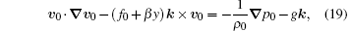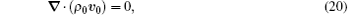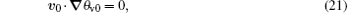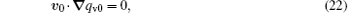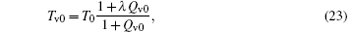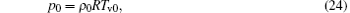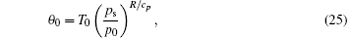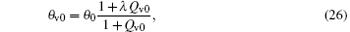where v0 = (u0, v0, 0). The thermal– wind relation can be derived as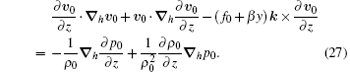Let (ue, ve, we, pe, Te, ρ e, θ e, θ ve, qve, qle, qe) denote the disturbances to the basic states, which is a function of x, y, z, and t. After neglecting the terms that are quadric and higher-order in disturbance amplitude, and reserving all of the source or sink and dissipations, one obtains the linearized disturbance equations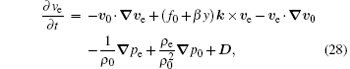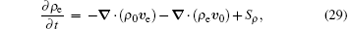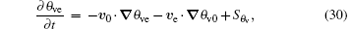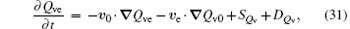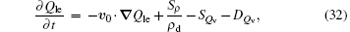in which ve = uei + vej + wek is the three-dimensional perturbation velocity and the Taylor series expansion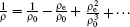has been used.

Under the assumption that | pe/p0| < 1, | ρ e/ρ 0| < 1, | Te/T0| < 1, | θ ve/θ v0| < 1, and | Qve/Qv0| < 1, one can linearize θ v and p by subtracting the basic-state equations  (24) and (26) from the state equation (15) and θ v, respectively. Consider the leading-order contributions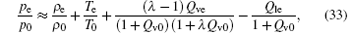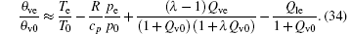Eliminating pe in Eqs.  (33) and (34) yields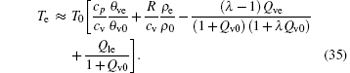As a disturbance to the basic-state VPV, q0 = ω 0 · θ v0/ρ 0 (ω 0 = (− ∂ v0/∂ z, ∂ u0/∂ z, ∂ v0/∂ x − ∂ u0/∂ y + f0 + β y)) is the basic-state absolute vorticity. The qe is given by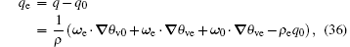where ω e = × ve is the perturbation relative vorticity. By performing a Taylor series expansion of C at q = q0 and θ v = θ v0, and considering the quadric contributions to C, one has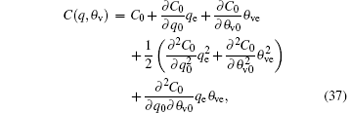where C0 = C(q0, θ v0) is the basic-state Casimir function.

We perform a Taylor series expansion of the quantity ρ (E + C(q, θ v)) after the substitution of u = u0 + ue, v = v0 + ve, w = we, Tv = Tv0 + Tve, q = q0 + qe, θ v = θ v0 + θ ve. By eliminating Te and qe in the leading-order terms of the expansion with Eqs.  (35) and (36), and neglecting the cubic and higher-order contributions, we may rewrite the quantity as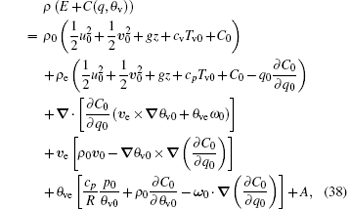where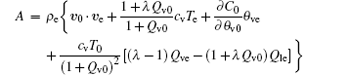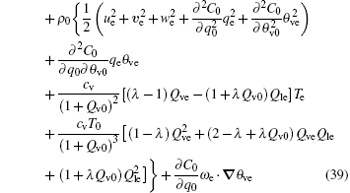is second-order in disturbance amplitude and is called the virtual pseudoenergy wave-activity density for a moist atmosphere. The basic-state Casimir function C0 = C(q0, θ v0) is the evaluation of C at q = q0 and θ v = θ v0.

Following Haynes and Ran et al., we can choose C0 to make the second, the fourth, and the fifth terms on the right-hand side of Eq.  (38) vanish. This requires C0 to satisfy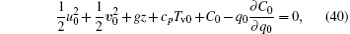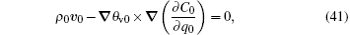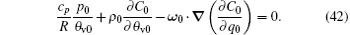The constraints (41) and (42) are trivially satisfied when equation  (40) is satisfied by the chain rule. It is easy to prove that equations (40)– (42) hold identically by taking the spacial partial derivatives of Eq.  (40) with respect to y and z to eliminate ∂ C0/∂ q0 in Eqs.  (41) and (42). The inherent consistency means that a solution of C0 to Eq.  (40) also satisfies Eqs.  (41) and (42).

By using Eqs.  (40)– (42), equation  (38) becomes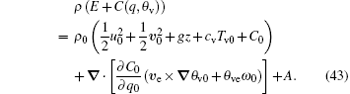Employing Eqs.  (40)– (42) again, equation  (43) is further simplified as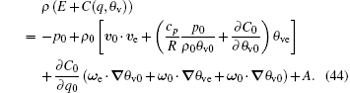After substituting Eq.  (43) into the first term on the left-hand side of Eq.  (17) and Eq.  (44) into the second term on the left-hand side of Eq.  (17), one may obtain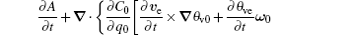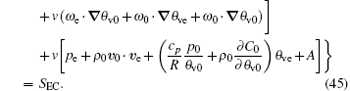Since the disturbance is assumed to be small-amplitude, the terms cubic in disturbance amplitude are neglected. Thus, the above equation may be rewritten as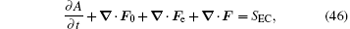where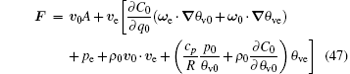is interpreted as the virtual pseudoenergy wave-activity flux, which is quadric in disturbance amplitude,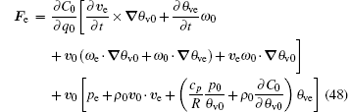is the leading-order perturbation flux, and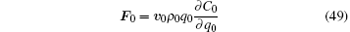is the basic-state flux.

It can be demonstrated that (see in Appendix A)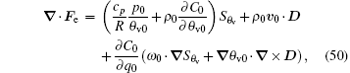and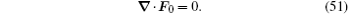Thus, the virtual pseudoenergy wave-activity relation may be obtained from Eq.  (46) as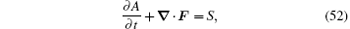where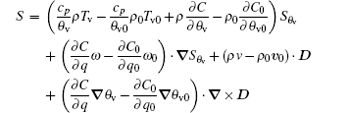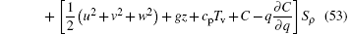is the source or sink caused by diabatic heating, dissipation, and mass forcing. Equation (52) shows that the virtual pseudoenergy wave-activity relation takes a local non-conservative form. Its non-conservation results from the diabatic heating, source, or sink and dissipation of qv, mass forcing, and momentum dissipation. For dry air, the water substance and mass forcing vanish so that θ v reduces to θ and equation (52) becomes the dry-air pseudoenergy wave-activity relation. If the forcing effects are not taken into account, then the dry-air pseudoenergy wave-activity is subjected to a local conservation form. If the moist atmosphere is unsaturated, which means there are not cloud hydrometeors and phase change of water substance, then the contribution of cloud hydrometeors to A in Eq.  (39) vanishes. Equation (52) is important at two points. One is that the relation is built in the non-hydrostatic and ageostrophic dynamic framework. This indicates that the relation is capable of diagnosing meso-scale systems. The other is that it involves water vapor and is applicable to the saturated and unsaturated moist atmosphere, which is not contained in other wave-activity theories.

In the practical application of Eq.  (52), how to calculate C is crucial, which may be overcome through two ways. One is that C0 is firstly calculated by numerically solving the integrated expression of C0 in Eq.  (40) with grid data, and then the known C0 is interpolated at q and θ v to evaluate C = C(q, θ v), just as Durran did. The other is to specify the expression of C in advance, such as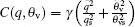in which γ is an arbitrary constant, qs and θ vs are the constant reference values of q and θ v, respectively. Then, the specified expression of C is brought into Eq.  (40), and is then combined with the basic-sated equations  (19)– (25) to evaluate the basic-state quantities with the given grid data by a numerical iteration method.

The wave-activity density represents some kind of wave energy and is an important variable in the wave– flow interaction theory. In order to validate the virtual pseudoenergy wave-activity density in relation (52), it is diagnosed in the following case study.

3. Case studies

This section focuses on two cases of heavy precipitation. One is a strong convection case which took place in the west of China on July 8, 2009, resulting in heavy rainfall. In this process, the precipitating system moved eastwards slowly in the belt of 36°   N– 39°   N. The other precipitation case occurred at 1200 UTC August 18, 2009– 0600 UTC August 19, 2009. In this case, the precipitation started in the north of Shanxi province and the middle of Inner Mongolia at 1200 UTC August 18, 2009 and then propagated northeastwards quickly. In these two cases, we examine the capabilities of virtual pseudoenergy wave-activity density A in diagnosing heavy rainfall, its physical meaning is also discussed.

3.1. Data

The grid analysis data are used here to do the case study and the related diagnosis. The data are obtained through the ARPS model. The national centers for environmental prediction (NECP) final analysis data and the routine observational data are read into the ARPS data assimilation system (ADAS) module, and then the objective analysis is conducted. The grid domain covers the area of North China (27.9°   N– 49.6°   N, 100°   E– 132°   E) with the center at (39.9°   N, 146.7°   E). The horizontal grids are 77 × 77 with the grid space 30  km in the zonal and meridional directions. The vertical depth is divided into 34 layers with the mean level space 500  m. The grid data are outputted on regular height levels at 6-h intervals.

3.2. Result

To analyze the integral characteristics of A in the troposphere, we calculate the vertical mean of A. Its horizontal distribution characteristics in the two events are examined. Figure  1 presents the horizontal distributions of A at 0000 UTC, 0600 UTC, 1200 UTC, and 1800 UTC July 8, 2009. It can be seen that in the whole heavy-rainfall process, the positive-value regions of A correspond well with the observed 6-h precipitation regions. The anomaly of A changes with the evolutions of the precipitating system or heavy-rainfall areas. What should be noticed is that A has a high positive value in the non-precipitation region because of its anomalous value in the upper troposphere. The negative-value A region is close to the positive-value A region. Thus, they form a fluctuating pattern. The precipitation is almost located in the positive amplitude region. Further analysis shows that the term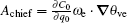is the chief composition of A (the figures are omitted due to space limitations). Since the term ∂ C0/∂ q0 is invariant with time, the term Achief mainly represents the temporal variation of the second-order potential vorticity (ω e · θ ve). The analysis also reveals that the term ω e · θ ve is mainly dominated by the barotropic component of perturbation PV (namely,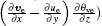). The perturbation θ ve increases with height in the mid-lower troposphere over the precipitation region, meaning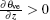. Moreover, there exists a strong perturbation of the cyclonic vorticity (namely,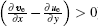) (the figures are omitted due to space limitations). The two factors are important dynamic and thermodynamic characteristics of precipitation. They present high values over the precipitation region, which further results in the positive anomalies of ω e · θ ve, Achief, and A. The anti-cyclonic vorticity perturbation (namely,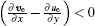) and the positive vertical gradient of θ ve give rise to the negative anomalies of ω e · θ ve, Achief, and A in the negative amplitude region.

 Figure Option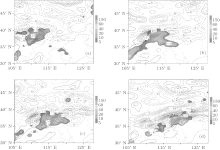Fig.  1. The virtual pseudoenergy wave-activity density (contour, 102  kg/(m · s2)) and the observed 6-h rainfall (shaded, mm) at (a) 0000 UTC July 8, (b) 0600 UTC July 8, (c) 1200 UTC July 8, and (d) 1800 UTC July 8, 2009.

 Figure Option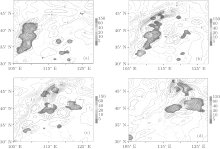Fig.  2. The virtual pseudoenergy wave-activity density (contour, 102  kg/(m · s2)) and the observed 6-h rainfall (shaded, mm) at (a) 1200 UTC August 18, (b) 1800 UTC August 18, (c) 0000 UTC August 19, and (d) 0600 UTC August 19, 2009.

The above analysis reveals that the virtual pseudoenergy wave-activity density with positive anomaly is capable of indicating precipitation regions in the studied event. To verifies this, another heavy-rainfall event during August 18, 2009– August 19, 2009 is further investigated. In this precipitation case, the positive anomalous region of A has a dominant correspondence to the strong precipitation. They both propagate northeastwards in an analogous phase. The consistency can also be explained by the fact that the vorticity perturbation is cyclonic and the virtual potential temperature perturbation increases vertically over the precipitation region.

4. Conclusions

In this paper, the energy-Casimir method used by Haynes is employed to derive the three-dimensional virtual pseudoenergy wave-activity relation for a moist atmosphere from the nonhydrostatic primitive equations in Cartesian coordinates. The three-dimensional basic states independent of time are chosen and the Casimir function, a monotonous function of virtual potential vorticity and virtual potential temperature, is introduced in the derivation. The virtual pseudoenergy wave-activity relation obtained here is characterized by taking into account the effects of water substances, mass forcing, diabatic heating, and dissipations. Constructed in the agoestrophic and nonhydrostatic dynamical framework, the virtual pseudoenergy wave-activity relation may be applicable to diagnose the evolution and propagation of meso-scale weather systems leading to heavy rainfall.

The virtual pseudoenergy wave-activity relation does not take a local conservation form due to diabatic heating, phase change of water vapor, mass forcing, and dissipations. Besides these forces, the divergence of the virtual pseudoenergy wave-activity flux contributes to the evolution of the virtual pseudoenergy wave-activity density. The relation is valid for dry, unsaturated, and saturated atmospheres.

The virtual pseudoenergy wave-activity density is further applied to two real cases of heavy precipitation. It is revealed that the virtual pseudoenergy wave-activity density distributes in a fluctuating pattern, including positive and negative anomaly regions. The positive anomaly is capable of indicating the precipitation region because it involves the cyclonic vorticity perturbation and vertically increasing virtual potential temperature perturbation. The two factors are important dynamic and thermodynamic characteristics of precipitation.

Reference
 1 Andrews D G 1983 J. Atmos. Sci. 40 1877 DOI:10.1175/1520-0469(1983)040<1877:AFAEPT>2.0.CO;2 [Cited within:1] [JCR: 2.672] 2 Andrews D G 1987 Q. J. R. Meteorol. Soc. 113 323 DOI:10.1002/qj.49711347518 [Cited within:1] 3 Andrews D G and McIntyre M E 1978 J. Fluid. Mech. 89 609 DOI:10.1017/S0022112078002773 [Cited within:1] [JCR: 1.415] 4 Huang R 1984 Adv. Atmos. Sci. 1 84 DOI:10.1007/BF03187619 [Cited within:1] [JCR: 1.338] [CJCR: 0.9244] 5 Magnusdottir G and Haynes P H 1996 J. Atmos. Sci. 53 2317 DOI:10.1175/1520-0469(1996)053<2317:WADATB>2.0.CO;2 [Cited within:1] [JCR: 2.672] 6 Murray D M 1998 J. Atmos. Sci. 55 2261 DOI:10.1175/1520-0469(1998)055<2261:APCLFT>2.0.CO;2 [Cited within:1] [JCR: 2.672] 7 Ren S 2000 J. Atmos. Sci. 57 3388 DOI:10.1175/1520-0469(2000)057<3388:FAWAIA>2.0.CO;2 [Cited within:1] [JCR: 2.672] 8 Scinocca J F and Peltier W R 1994 J. Atmos. Sci. 51 613 DOI:10.1175/1520-0469(1994)051<0613:FAWADF>2.0.CO;2 [Cited within:1] [JCR: 2.672] 9 Scinocca J F and Peltier W R 1994 J. Atmos. Sci. 51 623 DOI:10.1175/1520-0469(1994)051<0623:TIOLSS>2.0.CO;2 [Cited within:1] [JCR: 2.672] 10 Shepherd T G 1990 Adv. Geophys. 32 287 DOI:10.1016/S0065-2687(08)60429-X [Cited within:1] [JCR: 3.333] 11 Scinocca J F and Shepherd T G 1992 J. Atmos. Sci. 49 5 DOI:10.1175/1520-0469(1992)049<0005:NWACLA>2.0.CO;2 [Cited within:3] 12 Haynes P H 1988 J. Atmos. Sci. 45 2352 DOI:10.1175/1520-0469(1988)045<2352:FDGOFA>2.0.CO;2 [Cited within:4] [JCR: 2.672] 13 Boyd J P 1976 J. Atmos. Sci. 33 2285 DOI:10.1175/1520-0469(1976)033<2285:TNOWWT>2.0.CO;2 [Cited within:1] [JCR: 2.672] 14 Brunet G and Haynes P H 1996 J. Atmos. Sci. 53 482 DOI:10.1175/1520-0469(1996)053<0482:LLRORW>2.0.CO;2 [Cited within:1] [JCR: 2.672] 15 Durran D R 1995 J. Atmos. Sci. 52 3997 DOI:10.1175/1520-0469(1995)052<3997:PDFTDS>2.0.CO;2 [Cited within:1] [JCR: 2.672] 16 Zhang K and Feng J 2005 Acta Phys. Sin. 54 2985 118.145.16.217/magsci/article/article?id=17385598(in Chinese) [Cited within:1] [JCR: 1.016] [CJCR: 1.691] 17 McIntyre M E and Shepherd T G 1987 J. Fluid. Mech. 181 527 DOI:10.1017/S0022112087002209 [Cited within:1] [JCR: 1.415] 18 Ran L and Gao S 2007 J. Atmos. Sci. 64 2126 DOI:10.1175/JAS3931.1 [Cited within:2] [JCR: 2.672] 19 Ran L and Boyd J P 2008 Chin. Phys. B 17 1138 DOI:10.1088/1674-1056/17/3/065 [Cited within:1] [JCR: 1.148] [CJCR: 1.2429] 20 Ran L and Chu Y 2009 Acta Phys. Sin. 58 8094 118.145.16.217/magsci/article/article?id=17389705(in Chinese) [Cited within:1] 21 Zhou Y and Ran L 2010 Acta Phys. Sin. 59 1366 118.145.16.217/magsci/article/article?id=17391359(in Chinese) [Cited within:1] [JCR: 1.016] [CJCR: 1.691] 22 Ran L, Gao S and Cao J 2014 Chin. Phys. B 23 019201 DOI:10.1088/1674-1056/23/1/019201 [Cited within:1] [JCR: 1.148] [CJCR: 1.2429] 23 Shen X, Qing T and Li X 2013 Chin. Phys. B 22 094213 DOI:10.1088/1674-1056/22/9/094213 [Cited within:1] [JCR: 1.148] [CJCR: 1.2429] 24 Jiang Z, Li X, Zhou Y and Gao S 2012 Chin. Phys. B 21 054215 DOI:10.1088/1674-1056/21/5/054215 [Cited within:1] [JCR: 1.148] [CJCR: 1.2429] 25 Gao S, Li X and Zhou Y 2014 Chin. Phys. B 23 024204 DOI:10.1088/1674-1056/23/2/024204 [Cited within:1] [JCR: 1.148] [CJCR: 1.2429] 26 Ran L, Yang W and Chu Y 2010 Chin. Phys. B 19 079201 DOI:10.1088/1674-1056/19/7/079201 [Cited within:1] [JCR: 1.148] [CJCR: 1.2429] 27 Ran L, Zhou Y and Yang W 2011 Acta Phys. Sin. 60 099201 118.145.16.217/magsci/article/article?id=17393660(in Chinese) [Cited within:1] [JCR: 1.016] [CJCR: 1.691] 28 Chu Y, Wang Z and Ran L 2013 Acta Phys. Sin. 62 099201 DOI:10.7498/aps.62.099201(in Chinese) [Cited within:1] [JCR: 1.016] [CJCR: 1.691] 29 Skamarock W C, Klemp J B, Dudhia J, Gill D O, Barker D M, Wang W and Powers J G 2005 J. Comput. Phys. 227 3465 DOI:10.1016/j.jcp.2007.01.037 [Cited within:1] [JCR: 2.138] 30 Xue M, Droegemeier K K, Wong V, Shapiro A, Brewster K, Carr F, Weber D, Liu Y and Wang D 2001 Meteorol. Atmos. Phys. 76 143 DOI:10.1007/s007030170027 [Cited within:1] [JCR: 1.327] 31 Li X, Sui C, Lau K and Chou M 1999 J. Atmos. Sci. 56 3028 DOI:10.1175/1520-0469(1999)056<3028:LSFACR>2.0.CO;2 [Cited within:1] 32 Qiu C J and Xu Q 1992 J. Atmoc. Sci. 9 588 DOI:10.1175/1520-0426(1992)009<0588:ASAMOW>2.0.CO;2 [Cited within:1] 33 Gao S, Zhou Y, Cui X and Dai G 2004 Advance in Atmospheric Sciences 21 923 DOI:10.1007/BF02915594 [Cited within:1] [JCR: 1.338] [CJCR: 0.9244]